## Exploratory graphs¶

In :
import pandas as pd
import numpy as np


Here we'll experiment with different types of plots for the purpose of exploratory analysis. The purpose of exploratory analysis is mainly for ourselves to understand some basic structures of the data, and not to communicate results to other people. So we'll not concerned so much about legends, axes labels, etc.

It is soon clear to me that plotting in Python (via matplotlib) has a totally different paradigm from plotting in R. In matplotlib we often need to access the properties of the various components making up a plot, i.e. axes, labels, legend, or the plot points themselves, in order to make a nice, informative plot. This has the advantage that we know exactly what we're doing when plotting something.

R, on the other hand, often provides default plots for many different objects. E.g. we can just launch plot on almost any object, and something will be plotted. This makes a lot of things easy and gives often succinct plotting syntax, but on the other hand it is sometimes not entirely clear what is going on and what exactly is being plotted.

First let's load the data provided from the course's Dropbox URL:

In :
#pData = pd.read_csv('https://dl.dropbox.com/u/7710864/data/csv_hid/ss06pid.csv')
pData = pd.read_csv('../data/ss06pid.csv')
pData

Out:
<class 'pandas.core.frame.DataFrame'>
Int64Index: 14931 entries, 0 to 14930
Columns: 240 entries, Unnamed: 0 to pwgtp80
dtypes: float64(74), int64(163), object(3)

Explore different types of plots

In :
# pandas boxplot
pData.boxplot(column='AGEP');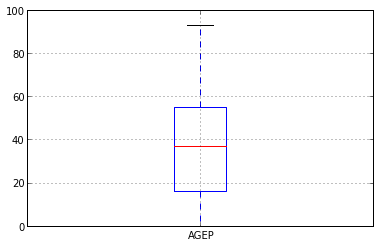In :
# pandas boxplot grouped by certain column
# setting width and axis names is rather tricky
pData.boxplot(column='AGEP', by='DDRS');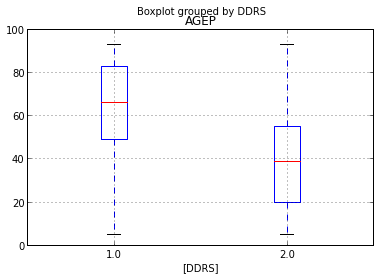In :
# pandas barplot
pData['CIT'].value_counts().plot(kind='bar');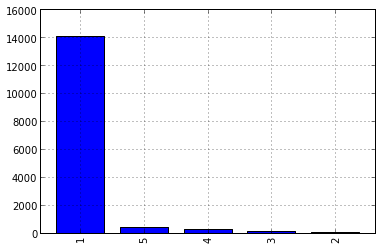In :
# pandas histogram plot
pData['AGEP'].hist(bins=18);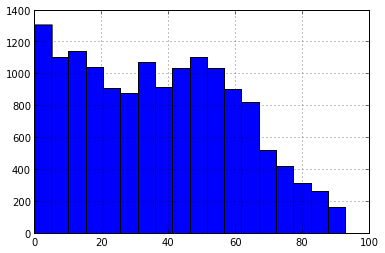In :
# pandas histogram plot with more bins
pData['AGEP'].hist(bins=100); plt.title('Age');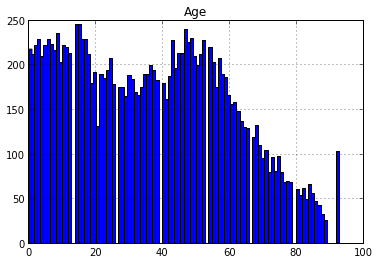In :
# pandas density plot
pData['AGEP'].plot(kind='kde', linewidth=3);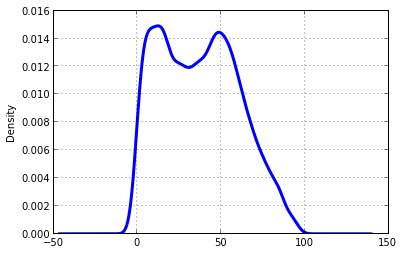In :
# pandas density plot, multiple distributions
pData['AGEP'].plot(kind='kde', linewidth=3);
pData['AGEP'][pData['SEX'] == 1].plot(kind='kde', linewidth=3, style='orange');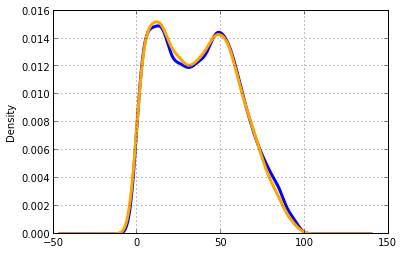In :
# pandas 'scatter' plot
pData.plot(x='JWMNP', y='WAGP', style='o');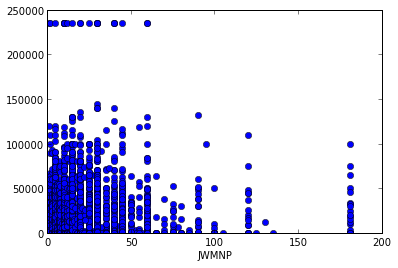In :
# scatterplot -- size matters
pData.plot(x='JWMNP', y='WAGP', style='o', markersize=3);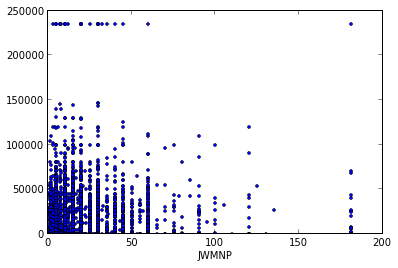In :
# scatterplot using colours
# here I switch to generic matplotlib plotting to be more flexible on styles
scatter(pData['JWMNP'], pData['WAGP'], c=pData['SEX'], s=15, cmap='autumn');
xlim(0,200)
ylim(0, 250000)
xlabel('JWNMP')
ylabel('WAGP');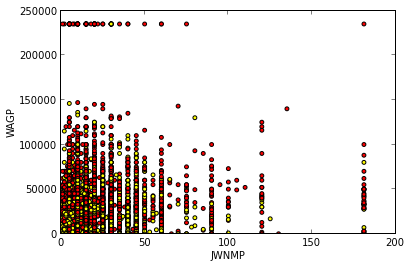In :
# scatterplots using size -- hard to see
percentMaxAge = pData['AGEP'].astype(float) / pData['AGEP'].astype(float).max()

scatter(pData['JWMNP'], pData['WAGP'], s=percentMaxAge*0.5);
xlim(0,200)
ylim(0, 250000)
xlabel('JWNMP')
ylabel('WAGP');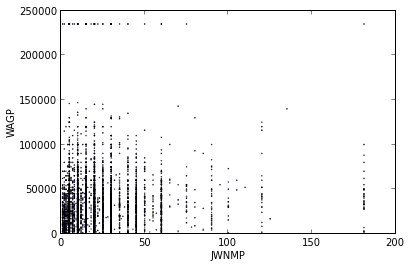In :
# scatterplots -- overlaying lines/points
scatter(pData['JWMNP'], pData['WAGP'], s=15)
xlim(0,200)
ylim(0, 250000)
xlabel('JWNMP')
ylabel('WAGP')

plot(np.repeat(100, pData.shape), pData['WAGP'], 'grey', linewidth=5)

plot(np.linspace(0, 200, num=100), np.linspace(0, 20e5, num=100), 'ro', markersize=10);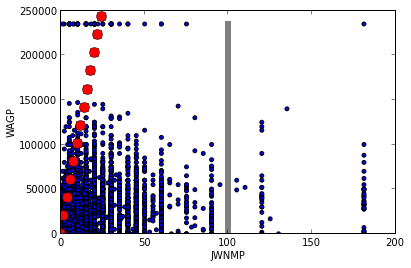Scatterplots with numeric variables as factors

So far almost all the plots are created with one line of code plus some lines to customize axes labels, ranges, etc. To do the plotting as below, we'll have to take a different approach. Namely the one plot produced below is actually achieved by running the scatter plot command several times in a for-loop.

The resulting code to produce the plot may look more verbose (i.e. longer) compared to R code doing the same thing, but as mentioned above, it's really clearer what the plot commands are doing.

In :
# scatterplots -- numeric variables as factors
ageGroups = pd.qcut(pData['AGEP'], 5)
pData['ageGroups'] = ageGroups.labels

cols = ['b', 'r', 'g', 'm', 'y']

i = 0
for k, df in pData.groupby('ageGroups'):
scatter(df['JWMNP'], df['WAGP'], c=cols[i], label=ageGroups.levels[k], alpha=.6)
i += 1

legend()
xlim(-2, 200)
ylim(0, 250000)
xlabel('JWMNP')
ylabel('WAGP');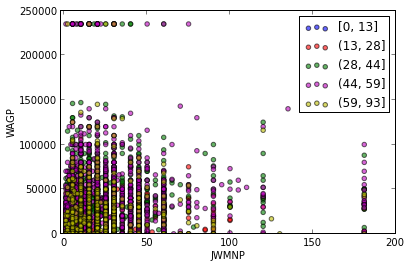Plotting lots of points

Suppose we have lots of points:

In :
x = np.random.normal(size=1e5)
y = np.random.normal(size=1e5)
plot(x, y, 'o');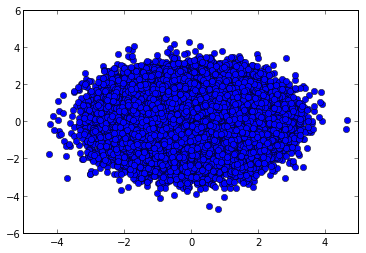The approach to perform plotting with so many points could be to pick some random samples:

In :
# a lot of points -- sampling
import random

sampledValues = random.sample(np.arange(1e5), 1000)
plot(x[sampledValues], y[sampledValues], 'o');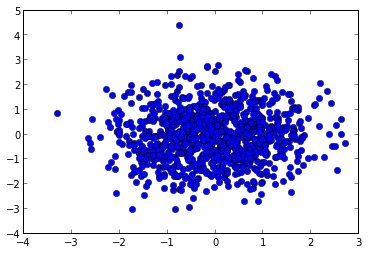Another approach is to use the so-called smoothscatter plot. No similar function in Python, so let's just nuke it using rpy2.

In :
%load_ext rmagic

In :
%Rpush x y

In :
%R smoothScatter(x, y)

KernSmooth 2.23 loaded
Copyright M. P. Wand 1997-2009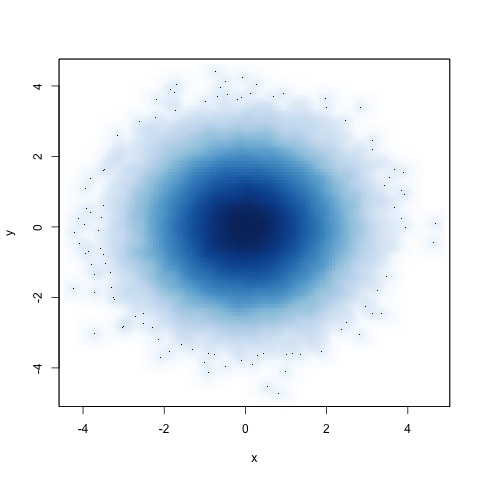Yet another different approach to plot lots and lots of points is to use hexbin.

In :
# a lot of points -- hexbin
hexbin(x, y);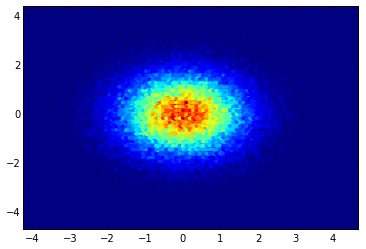qq-plot

Compare the distribution of the data with some reference distributions:

In :
# qq-plots is available in statsmodels
from statsmodels.graphics.gofplots import qqplot

x = np.random.normal(size=20)
y = np.random.normal(size=20)

# note: it seems like it's only possible to plot against distributions in scipy.stats.distributions (by default: normal)
# (i.e. not against a distribution of another variable)
qqplot(x, line='45', fit=True);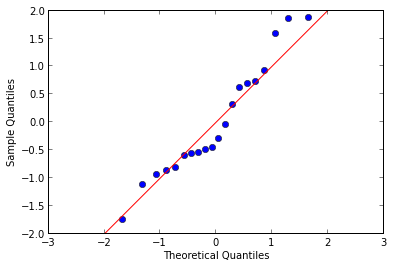More plots

In :
# spaghetti plot
X = np.array(np.random.normal(size=(20, 5)))

# there's no automatic cycle of markers
# but it's possible to do in matplotlib
# see: http://stackoverflow.com/questions/7358118/matplotlib-black-white-colormap-with-dashes-dots-etc
plot(X);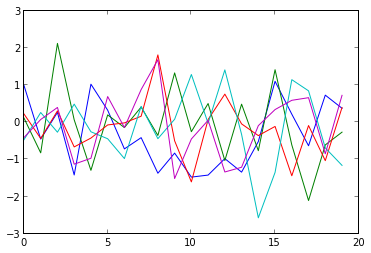In :
# 'heatmaps'
matshow(pData.ix[0:10, 161:237], aspect='auto', cmap='hot');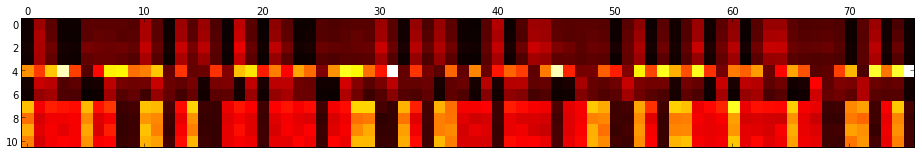In :
# maps
from mpl_toolkits.basemap import Basemap

figsize(9, 15)

m = Basemap()
m.drawcoastlines();
m.drawcountries();

lon = np.random.uniform(-180, 180, 40)
lat = np.random.uniform(-90, 90, 40)

m.plot(lon, lat, 'o');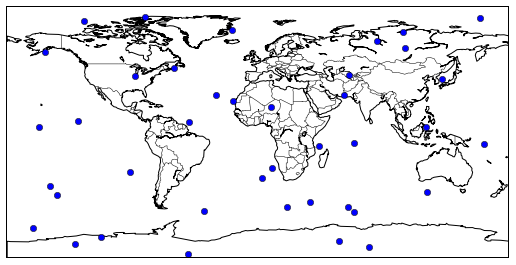In :
# missing values and plots
x = np.array([NaN, NaN, NaN, 4, 5, 6, 7, 8, 9, 10])
y = np.arange(1, 11)

figsize(7, 5)
plot(x, y, 'o');
xlim(0, 11); ylim(0, 11);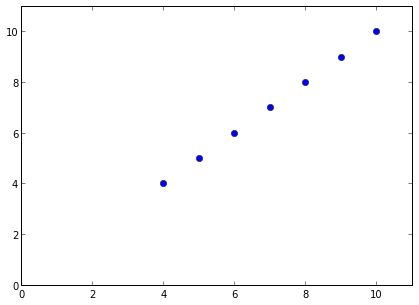In :
# missing values and plots
x = np.random.normal(size=100)
y = np.random.normal(size=100)

y[x < 0] = NaN

tt = pd.DataFrame(zip(x, np.isnan(y)), columns=['x', 'isnan y'])

tt.boxplot(column='x', by='isnan y');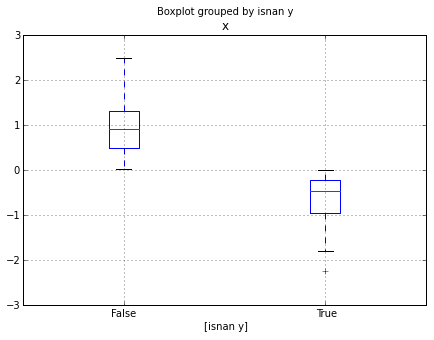In [ ]: